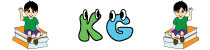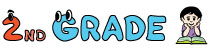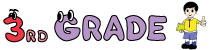1. Home>
2. Math>
3. Math Quiz>
4. Subtraction Quiz

# Subtraction Quiz - Online Subtraction Practice

These online subtraction quizzes provide excellent practice for kids from kindergarten through 3rd grade. The quizzes are based on horizontal or vertical subtraction; borrowing or no borrowing; finding the missing numbers; completing the subtraction sentences and many more. Subtraction drills are also provided for additional practice.Kindergarten subtraction test provides practice on horizontal and vertical subtraction restricting numbers up to 5 or 10. They can also try other quizzes on identifying missing numbers, finding the subtrahend and many more.First grade kids can get sufficient practice on subtracting numbers up to 20. Kids should find the difference up to 9 in both horizontal and vertical subtraction problems. Subtraction drills, balancing equation and more are also included.Second grade tests contain two-digit subtraction problems. Children can practice applying borrowing (regrouping) and no borrowing (no regrouping). The subtraction drills randomize 10 out of 25 problems and also 25 out of 50 problems.These subtraction quizzes are selectively provided for third grade children. They could work on these subtraction tests where 2-digit subtrahends are subtracted from 3-digit minuends. Additionally, three-digit subtraction drills are included.